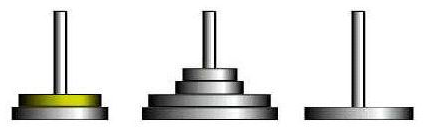시간 제한메모리 제한제출정답맞힌 사람정답 비율
1 초 128 MB2721239045.226%

## 문제

Charlie Darkbrown sits in another one of those boring Computer Science lessons: At the moment the teacher just explains the standard Tower of Hanoi problem, which bores Charlie to death!Figure 4: The standard (three) Towers of Hanoi.

The teacher points to the blackboard (Fig. 4) and says: "So here is the problem:

• There are three towers: A, B and C.
• There are n disks. The number n is constant while working the puzzle.
• All disks are different in size.
• The disks are initially stacked on tower A increasing in size from the top to the bottom.
• The goal of the puzzle is to transfer all of the disks from tower A to tower C.
• One disk at a time can be moved from the top of a tower either to an empty tower or to a tower with a larger disk on the top.

So your task is to write a program that calculates the smallest number of disk moves necessary to move all the disks from tower A to C."

Charlie: "This is incredibly boring—everybody knows that this can be solved using a simple recursion.I deny to code something as simple as this!"

The teacher sighs: "Well, Charlie, let's think about something for you to do: For you there is a fourth tower D. Calculate the smallest number of disk moves to move all the disks from tower A to tower D using all four towers."

Charlie looks irritated: "Urgh. . . Well, I don't know an optimal algorithm for four towers. . . "

So the real problem is that problem solving does not belong to the things Charlie is good at. Actually, the only thing Charlie is really good at is "sitting next to someone who can do the job". And now guess what — exactly! It is you who is sitting next to Charlie, and he is already glaring at you.

Luckily, you know that the following algorithm works for n <= 12: At first k >= 1 disks on tower A are fixed and the remaining n-k disks are moved from tower A to tower B using the algorithm for four towers.Then the remaining k disks from tower A are moved to tower D using the algorithm for three towers. At last the n - k disks from tower B are moved to tower D again using the algorithm for four towers (and thereby not moving any of the k disks already on tower D). Do this for all k 2 ∈{1, .... , n} and find the k with the minimal number of moves.

So for n = 3 and k = 2 you would first move 1 (3-2) disk from tower A to tower B using the algorithm for four towers (one move). Then you would move the remaining two disks from tower A to tower D using the algorithm for three towers (three moves). And the last step would be to move the disk from tower B to tower D using again the algorithm for four towers (another move). Thus the solution for n = 3 and k = 2 is 5 moves. To be sure that this really is the best solution for n = 3 you need to check the other possible values 1 and 3 for k. (But, by the way, 5 is optimal. . . )

## 입력

There is no input.

## 출력

For each n (1 <= n <= 12) print a single line containing the minimum number of moves to solve the problem for four towers and n disks.

## 채점 및 기타 정보

• 예제는 채점하지 않는다.